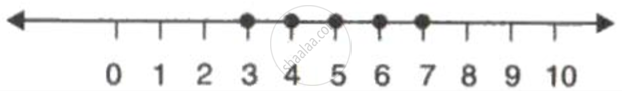Advertisement Remove all ads

# If P = { X : -3 < X ≤ 7, X ∈ R} and Q = { X : - 7 ≤ X < 3, X ∈ R} , Represent the Following Solution Set on the Different Number Lines: - Mathematics

Sum

If P = { x : -3 < x ≤ 7, x ∈ R} and Q = { x : - 7 ≤ x < 3, x ∈ R} , represent the following solution set on the different number lines:

P-Q

Advertisement Remove all ads

#### Solution

P = { x : -3 < x ≤ 7, x ∈ R} and Q = { x : - 7 ≤ x < 3, x ∈ R}

p = {-2, -1, 0, 1, 2, 3, 4, 5, 6, 7}  and Q = {-7, -6, -5, -4, -3, -2, - 1, 0, 1, 2}

P-Q = {3,4,5,6,7}Concept: Solving Algebraically and Writing the Solution in Set Notation Form
Is there an error in this question or solution?
Advertisement Remove all ads

#### APPEARS IN

Frank ICSE Class 10 Mathematics Part 2
Chapter 5 Linear Inequations
Exercise 5.1 | Q 14.3 | Page 75
Advertisement Remove all ads
Advertisement Remove all ads
Share
Notifications

View all notifications

Forgot password?
Course